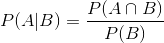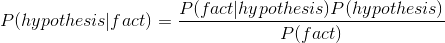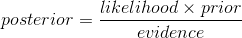#2017 iT 邦幫忙鐵人賽
DAY 9
0
Big Data

# 條件機率# 貝式定理1. `P(fact|hypothesis)`意思是假設某個假說是對的，那我手上的資料有多麼符合這個假說，也就是可能性的意思
2. `P(fact)`這邊代表的是先驗機率(prior probability)，這個地方比較特別，他需要你把預先知道的知識放進去一起估計# 貝式推論框架

``````P(抽出一顆黃色|H1成立) = C5取1 * 0.1 * 0.9^4 = 0.32805
P(抽出一顆黃色|H2成立) = C5取1 * 0.2 * 0.8^4 = 0.4096
....
P(抽出一顆黃色|H9成立) = C5取1 * 0.9 * 0.1^4 = 0.00045
``````

``````P(H1成立|抽出一顆黃色) = (0.32805 * 1/9) / 各項分子的總和
.....
``````

0